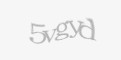Friday, 28 April 2023 07:50

## PLAY WITH OHM

Rate this item
|||||| ||||||

Calculate a resistor value and its current flow

Learn how to apply a Voltage Divider and an Arduino to find the value of an unknow resistor. Then, using the Ohm Law, calculate the amount of the current flow.

• What you will learn:
• Science
• - application of Ohm laws
• - application of the Voltage Divider
• Math:
• - practice with the calculation related to the applied laws
• Engineering
• - wire electronic circuit following a simple diagram
• Technology:
• - arduino programming using analog signals, float variables and math operators
• Expected Time:

2-5 h

• Materials & Recycled Materials:
• - 1x Arduino board (e.g. Arduino Uno)
• - 1x 100Ω resistor. Power of the resistor 1/4W or more
• - Dupont Cables
• - Different type of resistors (R)
• Guide:
• 1. Following the available electrical diagram, connect the 100Ω resistor to the Arduino Board (it will be called "Fixed Resistor"). Choose a random resistor (you can cath up it from old electronic boards). Try to calculate what will be the expected Resistance and Current results.
• 2. Declare the needed variables as floating type so you can manage easily decimal numbers. You need to have variables to declare the measured values (Supply voltage and Fixed resistor voltage, those are raw values from the analog input) , and the calculated values (Supply converted voltage, Fixed resistor converted voltage, Value of the fixed resistor, Calculated resistor result, Circuit current value). In this exercise we suggest to measure the supply voltage (in theory it is 5 volts) in order to be more accurated.
• 3. In the Setup, using the Serial.begin command, start the serial communication in order to print whatever you need on the serial monitor
• 4. In the Loop, at first you need to read the Voltages from the analog input. We suggest to use a For cycle in order to read the value several time, then calculate the average in order to reduce floating errors.
• 5. Apply then the Voltage Divisor formula to obtain the value of your resistor
• 6. Apply also the Ohm's law to obtain the value of the current flow trought the circuit
• 7. Using print commands, write on the Serial Monitor the results
• 8. Test the functionality of your program, comparing the practical results with the calculated ones
• 9. Try to change the resistor with a different one and check the accuracy of the results.
• Further Steps:

If you want more fun you can:

• - Use external voltage supplier (e.g. a 1.5 Volts battery)
• - Use voltage and and current sensor
• - Use a display and build your ohm meter!
• Software used in the project:
• - Arduino IDE
• Difficulty level: Easy
• Developed By: Apro Formazione - IT

### Media

No media available for this project